Category: Forgotten Mathematics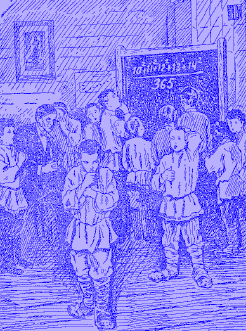Let us say somebody ask you to solve the next equation without using any technical aid.

Can you do this?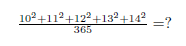O.k. it is not so easy. But when you know the special and interesting relation between those numbers:it is simple. The left components of the equation are: 100 + 121 + 144 = 365; In other words: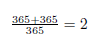O.k. let us figure out with the aid of little algebra if we can find more of such sequences: The first number we are looking for is “x”: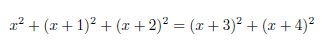or simple: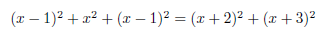The equation can be written as quadratic equation: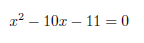The solution is: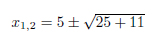In fact we have two solutions: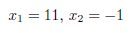It means we have two sequence with considered behavior. The first one, has been already checked by (10,11,12,13,14). The new one is: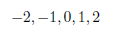Let us check it: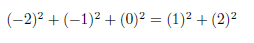It works!